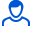Positive responses: 2
Negative responses: 0

Refunds: 0Topics formulas:

a linear algebra (matrices, determinants) + solutions to

-trigonometriya

-limit

-integrals (+ ways to solve)

-formula abridged multiplication

-derivatives

-vectors (in the plane and in space)

-surfaces second order

operation with vectors

-All of complex numbers

-...

In addition, the page with the formulas is divided into small squares, which spur is cut and folded in an accordion.
Himself with this spur exam :)
No feedback yet